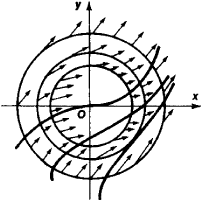# Direction Field

The following article is from The Great Soviet Encyclopedia (1979). It might be outdated or ideologically biased.

## Direction Field

a set of points in the xy-plane such that at each point a certain direction is defined. The direction is usually represented by an arrow passing through the point.

If the equation = f(x, y) is given, then at each point (x0, y0) of some region in the xy-plane the value of the slope k = f (x0, y0) of the tangent to the integral curve passing through this point is known, so that the direction of the tangent can be represented by an arrow. Thus the differential equation defines a direction field; conversely, a direction field in some region of the xy-plane defines a differential equation of the form = f(x, y). By sketching sufficiently many isoclines (curves joining points at which the direction field f (x, y) = C, C a constant, has the same value), we can obtain approximations to the integral curves, that is, curves having the prescribed directions as tangents (the isocline method).Figure 1

Thedirection field of the equation = x2 + y2 is shown in Figure 1. The light curves (circles) represent isoclines, and the thicker curves represent integral curves.

### REFERENCES

Stepanov, V. V. Kurs differentsial’nykh uravnenii, 8th ed. Moscow, 1959.
Petrovskii, I. G. Lektsii po teorii obyknovennykh differentsial’nykh uravnenii, 6th ed. Moscow, 1970.